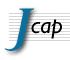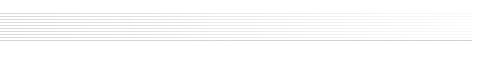## Keyword List

 Note The keywords are organized in sections. The section name is highlighted in grey followed by the list of individual keywords belonging to that section.

 Group: JCAP Keywords
Methods for astroparticle physics and cosmology Astrophysical plasma and magnetic fields
• Bayesian reasoning
• Frequentist statistics
• Machine learning
• Statistical sampling techniques
• astrophysical fluid dynamics
• cosmic magnetic fields theory
• extragalactic magnetic fields
• galactic magnetic fields
• magnetic fields
• Magnetohydrodynamics
• millisecond pulsars
• pulsar magnetosphere
• solar physics
• X-ray pulsar
Cosmic Microwave Background Radiation Dark matter and dark energy
• CMBR detectors
• CMBR experiments
• CMBR polarisation
• CMBR theory
• cosmological parameters from CMBR
• gravitational waves and CMBR polarization
• integrated Sachs-Wolfe effect
• non-gaussianity
• reionization
• Sunyaev-Zeldovich effect
• cluster counts
• dark energy experiments
• dark energy theory
• dark matter detectors
• dark matter experiments
• dark matter simulations
• dark matter theory
• galaxy clustering
• rotation curves of galaxies
• supernova type Ia - standard candles
• weak gravitational lensing
Early Universe Galaxies
• alternatives to inflation
• axions
• baryon asymmetry
• big bang nucleosynthesis
• cosmic singularity
• Cosmic strings, domain walls, monopoles
• cosmological applications of theories with extra dimensions
• cosmological perturbation theory
• cosmological phase transitions
• cosmology of theories beyond the SM
• cosmology with extra dimensions
• extra dimensions
• inflation
• Inflation and CMBR theory
• initial conditions and eternal universe
• leptogenesis
• particle physics - cosmology connection
• physics of the early universe
• primordial black holes
• primordial magnetic fields
• quantum cosmology
• quantum gravity phenomenology
• recombination
• string theory and cosmology
• supersymmetry and cosmology
• transplanckian physics
• dwarfs galaxies
• feedback in galaxies
• galaxy dynamics
• galaxy evolution
• galaxy formation
• galaxy morphology
• galaxy surveys
• high redshift galaxies
• hydrodynamical simulations
• massive black holes
• semi-analytic modeling
Gravity High energy astrophysics
• Cosmological perturbation theory in GR and beyond
• Equations of motion, and 2-body problem in GR and beyond
• Exact solutions, black holes and black hole thermodynamics in GR and beyond
• Gauss-Bonnet-Lovelock-Horndeski-Palatini etc gravity theories
• GR black holes
• Gravastars
• gravitational wave detectors
• gravitational waves / experiments
• gravitational waves / sources
• gravitational waves / theory
• Gravitational waves in GR and beyond : theory
• gravity
• modified gravity
• primordial gravitational waves (theory)
• quantum black holes
• quantum field theory on curved space
• Quantum fields in curved spacetimes
• Wormholes
• X-ray telescopes
• accretion
• active galactic nuclei
• cosmic ray experiments
• cosmic ray theory
• cosmic rays detectors
• gamma ray burst experiments
• gamma ray bursts theory
• gamma ray detectors
• gamma ray experiments
• gamma ray theory
• particle acceleration
• ultra high energy cosmic rays
• X-ray binaries
• X-rays
Large scale structure of the universe Neutrinos
• baryon acoustic oscillations
• cosmic flows
• cosmic web
• cosmological parameters from LSS
• cosmological simulations
• galaxy clusters
• gravitational lensing
• intergalactic media
• Lyman alpha forest
• power spectrum
• redshift surveys
• superclusters
• cosmological neutrinos
• double beta decay
• neutrino astronomy
• neutrino detectors
• neutrino experiments
• neutrino masses from cosmology
• neutrino properties
• neutrino theory
• solar and atmospheric neutrinos
• supernova neutrinos
• ultra high energy photons and neutrinos
Stars
• astrophysical black holes
• core-collapse supernovae
• first stars
• massive stars
• neutron stars
• star explosions
• star formation
• stars
• supernova dynamics
• supernova evolution
• supernova remnants
• supernovas
• white and brown dwarfs